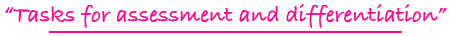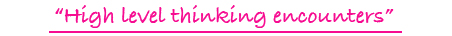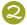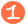•••Tiling Task Card Packets include 20 unique task cards on card stock, 3 backdrop cards, tracking sheets, suggestions and answer sheets.  These are contained in a printed manila envelope for convenient storing.  A set of number tiles 0 - 9 is  needed for each task card.

4 - 7

FRACTION & NUMBER LINE TILES

comparing common fractions ( )using a visual model of a number line between zero and one

Challenge Level:PDF FORMAT
(You will receive both color and black and white files for printing.)\$10.00

4 - 7

FRACTION COMPARISON TILES DIGITAL

comparing two fractions with different numerators and different denominators using visual models to record comparisons with symbols

Challenge Level:DIGITAL FORMAT\$10.00

4 - 7

FRACTION COMPARISON TILES

comparing two fractions with different numerators and different denominators using visual models to record comparisons with symbols

Challenge Level:PDF FORMAT
(You will receive both color and black and white files for printing.)\$10.00

4 - 8

FRACTION TILES DIGITAL

experimenting with number tiles to form common fractions; example: fractions equivalent to 3/4

Challenge Level:DIGITAL FORMAT\$10.00

4 - 8

FRACTION TILES

experimenting with number tiles to form common fractions; example: fractions equivalent to 3/4

Challenge Level:PDF FORMAT
(You will receive both color and black and white files for printing.)\$10.00

4 - 8

FRACTIONAL PART TILES

finding fractional parts of whole numbers; examples: 1/3 of 39; less than 1/4 of 100

Challenge Level:\$15.00

4 - 8

FRACTIONAL PART TILES DIGITAL

finding fractional parts of whole numbers; examples: 1/3 of 39; less than 1/4 of 100

Challenge Level:DIGITAL FORMAT\$10.00

4 - 8

FRACTIONAL PART TILES

finding fractional parts of whole numbers; examples: 1/3 of 39; less than 1/4 of 100

Challenge Level:PDF FORMAT
(You will receive both color and black and white files for printing.)\$10.00

5 - 8

MULTI-DECI TILES

multiplying decimal fractions

Challenge Level:\$15.00

5 - 8

PERCENTAGE TILES I

changing common fractions to decimals and decimals to percents and vice versa

Challenge Level:\$15.00

5 - 8

PERCENTAGE TILES II

computing the three basic types of percent problems

Challenge Level:\$15.00

3 - 6

PICTURE FRACTION TILES

forming common fractions from pictures, including equivalent fractions and geometry vocabulary for part of a total group

Challenge Level:\$15.00

3 - 6

PICTURE FRACTION TILES DIGITAL

forming common fractions from pictures, including equivalent fractions and geometry vocabulary for part of a total group

Challenge Level:DIGITAL FORMAT\$10.00

3 - 6

PICTURE FRACTION TILES

forming common fractions from pictures, including equivalent fractions and geometry vocabulary for part of a total group

Challenge Level:PDF FORMAT
(You will receive both color and black and white files for printing.)\$10.00

5 - 8

+/- DECI TILES

addition and subtraction of decimal fractions

Challenge Level:\$15.00

3 - 6

VIEW A FRACTION TILES DIGITAL

forming common fractions from pictures, including equivalent fractions and comparisons of fractions

Challenge Level:DIGITAL FORMAT\$10.00

3 - 6

VIEW A FRACTION TILES

forming common fractions from pictures, including equivalent fractions and comparisons of fractions

Challenge Level:PDF FORMAT
(You will receive both color and black and white files for printing.)\$10.00

5 - 8

VISUALIZING TILES

dealing with representational models for common fractions, percent, ratio, and square numbers

Challenge Level:\$15.00